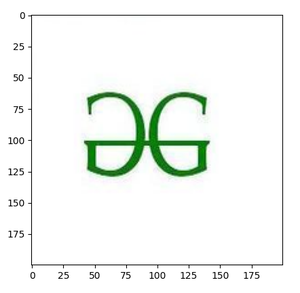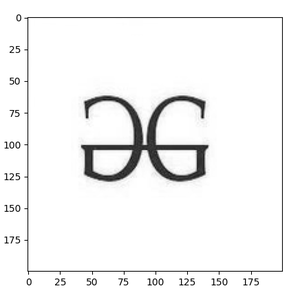How to Display an OpenCV image in Python with Matplotlib?

• Difficulty Level : Medium
• Last Updated : 16 Aug, 2021

The OpenCV module is an open-source computer vision and machine learning software library. It is a huge open-source library for computer vision, machine learning, and image processing. OpenCV supports a wide variety of programming languages like Python, C++, Java, etc. It can process images and videos to identify objects, faces, or even the handwriting of a human. When it is integrated with various libraries, such as numpy which is a highly optimized library for numerical operations, then the number of weapons increases in your Arsenal i.e whatever operations one can do in Numpy can be combined with OpenCV.

First, let’s look at how to display images using OpenCV:

Now there is one function called cv2.imread() which will take the path of an image as an argument. Using this function you will read that particular image and simply display it using the cv2.imshow() function.

Python3

 # import required moduleimport cv2 # read the Image by giving pathimage = cv2.imread('gfg.png') # display that imagecv2.imshow('GFG', image)

Output:DIsplay image using OpenCV

Now let’s jump into displaying the images with Matplotlib module. It is an amazing visualization library in Python for 2D plots of arrays. The Matplotlib module is a multi-platform data visualization library built on NumPy arrays and designed to work with the broader SciPy stack.

We are doing minor changes to the above code to display our image with Matplotlib module.

Python3

 # import required moduleimport cv2import matplotlib.pyplot as plt # read imageimage = cv2.imread('gfg.png') # call imshow() using plt objectplt.imshow(image) # display that imageplt.show()

Output:image plot with Matplotlib

One can also display gray scale OpenCV images with Matplotlib module for that you just need to convert colored image into a gray scale image.

Python3

 # import required modulesimport cv2import matplotlib.pyplot as plt # read the imageimage = cv2.imread('gfg.png') # convert color image into grayscale imageimg1 = cv2.cvtColor(image, cv2.COLOR_RGB2GRAY) # plot that grayscale image with Matplotlib# cmap stands for colormapplt.imshow(img1, cmap='gray') # display that imageplt.show()

Output:Display grayscale image plot with Matplotlib

This is how we can display OpenCV images in python with Matplotlib module.

My Personal Notes arrow_drop_up